Courses

# How to use Vernier Calipers? Class 11 Notes | EduRev

## Class 11 : How to use Vernier Calipers? Class 11 Notes | EduRev

The document How to use Vernier Calipers? Class 11 Notes | EduRev is a part of the Class 11 Course Physics Class 11.
All you need of Class 11 at this link: Class 11

VERNIER CALIPER
Definition: A caliper is a device used to measure the distance between two opposing sides of an object.
The tips of the caliper are adjusted to fit across the points to be measured and the caliper is then removed and the distance between the tips is measured using a ruler.
The modern Vernier caliper was invented by Joseph R. Brown in 1851.
The Vernier Caliper consists of a main scale fitted with a jaw at one end (figure). Another jaw, containing the Vernier scale, moves over the main scale (slidable). When the two jaws are in contact, the zero of the main scale and the zero of the Vernier scale should coincide. If both the zeros do not coincide, there will be an error called zero error.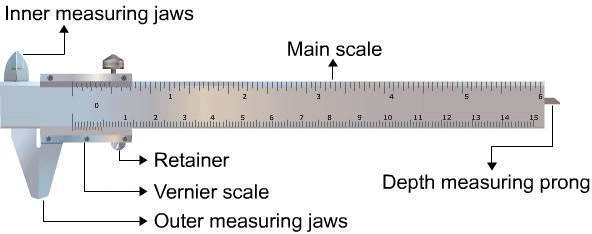Parts of a Vernier Caliper
1. Main Scale
The main scale consists of a steel metallic strip graduated in centimeters at one edge and in inches at the other edge . It carries the inner and outer measuring jaws.

2. Vernier Scale
A vernier scale  slides on the strip. It can be fixed in any position by the retainer. On the Vernier scale, generally, 0.9 cm is divided into ten equal parts.

3. Outer Measuring Jaws
The outer measuring jaws help to take the outer dimension of an object while the inner measuring jaws help to take the inner dimension of an object.

4. Retainer
The retainer helps to retain the object within the jaws of the Vernier calipers

5. Depth Measuring Prong
The depth measuring prong helps to measure the depth of an object

Concept of Least Count
The least count or the smallest reading which you can get with the instrument can be calculated as;
Least Count = One Main Scale division (MSD)-One Vernier Scale Division (VSD)
or
Least Count = (One  Main scale(MS) division/Number of divisions in Vernier Scale)

First calculate the least count and only then place the object between the two jaws.
Record the position of zero of the Vernier scale on the main scale.

When a body is between the jaws of the Vernier Caliper; firstly, we need to ensure that the zero of the main scale and the zero of the Vernier scale should coincide. If both the zeros do not coincide, there will be an error called zero error.
Now,
Observe the position of the zero on the Vernier Scale. If the zero of the Vernier scale lies ahead of the Nth division of the main scale, then the main scale reading (MSR) is;
MSR = N
If nth division of Vernier scale coincides with any division of the main scale, then the Vernier scale reading (VSR) is;
VSR = n × L.C, ( L.C is least count of vernier calliper)
Now, the total reading of the length is,
i.e
TR = MSR + VSR = N + (n × L.C) .....(2)

Finding volumes using Vernier Caliper
(a) Volume of a Beaker / Calorimeter
Volume of the beaker / calorimeter = internal area of the cross section x depth
This can be expressed as;
V = π(D/2)2.d
where 'D' is the internal diameter of beaker / calorimeter and 'd' the depth of beaker / calorimeter.
Now, readings of D and d which represents the diameter of cross section and depth respectively can be taken using the Vernier Caliper. The diameter can be measured by clamping the cross section area into the jaws while the depth can be measured using Depth Measuring Prong.

(b) Volume of a Sphere
V = (4/3)πr3
where 'r' is the radius of the sphere.
Measurement of r is done using the Vernier Caliper

(c) Volume of a Rectangular Block
V = l * b * h
where 'l' is length of the block, 'b' the breadth and 'h' the height of the block.

Materials Required

• Vernier callipers
• A spherical body (it can be a pendulum bob)
• A cylinder.
• A small rectangular metallic block of known mass
• A beaker or a calorimeter.

The Procedure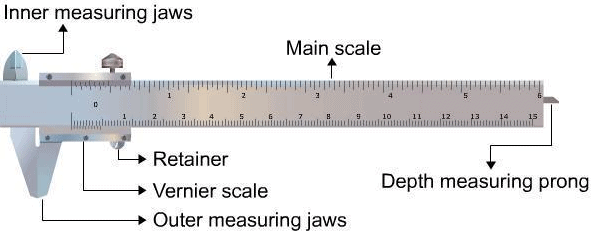The Procedure
(1) We'll first determine the vernier constant (VC), which is the least count (L.C) of the vernier calliper and record it stepwise as in the equation,
L.C = 1 MSD - 1 VSD.

(2) Now, bring the movable jaw in close contact with the fixed jaw and find the zero error. Do this three times and record the values. If there is no zero error, then record 'zero error nil'.

(3) Open the jaws of the Vernier Calliper and place the sphere or cylinder between the two jaws and adjust the movable jaw, such that it gently grips the body without any undue pressure on it. After that, tighten the screw attached to the Vernier scale (figure).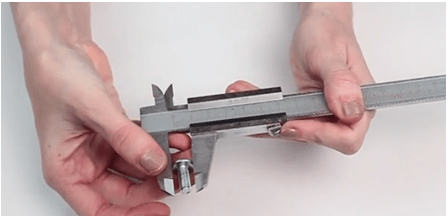(4) Note the position of the zero mark of the Vernier scale on the main scale. Record the main scale reading just before the zero mark of the Vernier scale. This reading (N) is called main scale reading (MSR).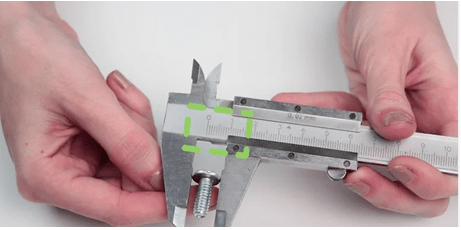(5) Note the number (n) of the Vernier scale division which coincides with the division of the main scale.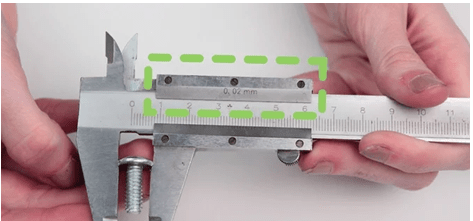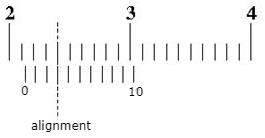(6) You'll have to repeat steps 5 and 6 after rotating the body by 90o for measuring the diameter in a perpendicular direction.

(7) Repeat steps 4 to 7 for at least three different positions and record the observations.

(8) Now find total reading using the equation, TR = MSR+VSR = N+(n x L.C)  and apply the zero correction.

(9) Take the mean of the different values of the diameter and show that in the result with the proper unit.

Note:
To measure the internal diameter of a calorimeter or beaker, place the beaker upside down over the internal jaws of the vernier calipers.Then repeat the steps 4 to 8.

To find the ‘Depth’ of the beaker, move the metallic strip till it touches the bottom of the beaker. Then repeat steps 4 to 8.

Recording our Observations(Table)
We can conduct any measurements using the following steps and record each value as given.
(a) Determination of Vernier constant (Least Count ) of the vernier callipers:
1 M.S.D. = 1 mm
10 V.S.D.= 9 M.S.D.
1 V.S.D.= 9/10 M.S.D. = 0.9 mm.
Hence, every division on a Vernier scale corresponds to a length of 0.9 mm.
Thus,
Vernier Constant, V.C.= 1 M.S.D.-1 V.S.D. = (1-0.9) mm = 0.1 mm = 0.01 cm.

Observe the least count of Vernier Caliper is 0.01 cm which is 10 times smaller than the general scale we use in daily life measurements.
Hence, we can conclude that measurements made using the Vernier Caliper are 10 times more accurate than normal scale.

(b) Zero error
(i).........cm,
(ii).........cm,
(iii)...........cm.
Mean zero error (e)=..........cm.
Mean zero correction (c) = -e =.........cm.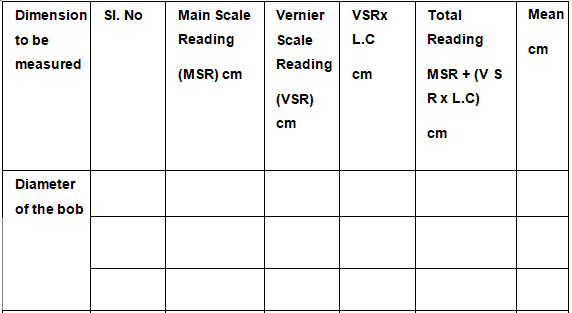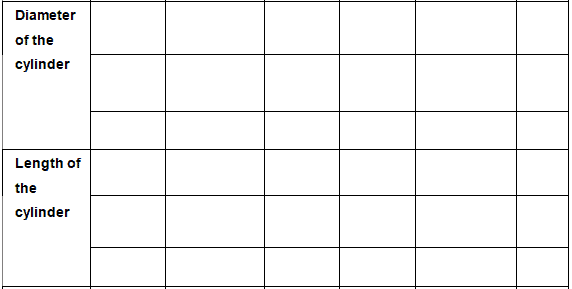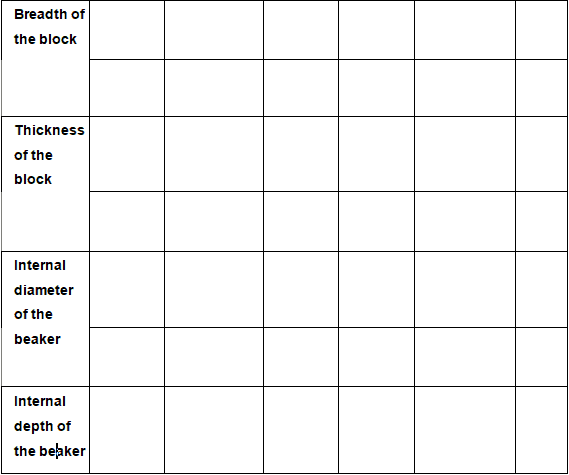Calculations
Mean corrected diameter------------cm
Volume of sphere, V= 4/3 π=---------cm3
= ------m3.
Mean corrected length of the block, l=............cm
Mean corrected breadth of the block,  b= .......cm
Mean corrected thickness of the block,  h= .........cm
Volume of block , L × B × H =........................cm3
=..........m3
Density of the block material, m/v=..................cm
Mean corrected internal diameter,D=................cm
Mean corrected depth,d=........cm
Volume of beaker / calorimeter, v = π(D/2)2 = ..........cm3
=............m3.

The Result
The volume of the beaker / calorimeter is ...........m3.
Volume of Sphere=.......................... m3
Volume of block is ................................m3
The volume of the  beaker / calorimeter is ...........cm3.

Solved Examples:
Q.1. What is the smallest possible reading (in cm) of a Vernier caliper?
Ans.The smallest possible reading of a Vernier caliper is 0.01 cm also called the Least Count of the scale (L.C.).

Q.2. A measurement on the Vernier Caliper is made. Report the length of the scale.
Ans.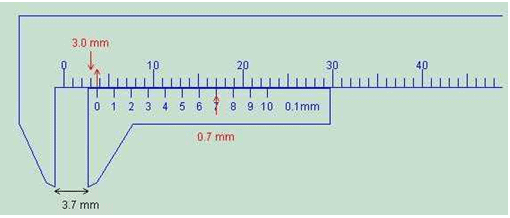Observe that the Main scale reading (MSR) =  3.0 mm
Also, the 7th division mark on the Vernier scale exactly coincides with the main scale. Hence, Vernier scale reading is 7.
TR = MSR + VSR = N + (n × L.C)   ..... (2)
TR = 3.0 mm + (7 × 0.1)mm = 3.7 mm
Hence, the reading recorded would be 3.7 mm.

Q.3. A measurement on the Vernier Caliper is made. Report the length of the scale.
Ans.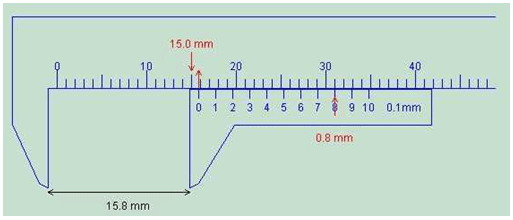Observe that the Main scale reading (MSR) = 15.0 mm
Also, the 8th division mark on the Vernier scale exactly coincides with the main scale. Hence, Vernier scale reading is 8.
TR = MSR + VSR = N + (n × L.C)  ..... (2)
TR = 15.0 mm + (8 × 0.1)mm = 15.8 mm
Hence, the reading recorded would be 15.8 mm.

Offer running on EduRev: Apply code STAYHOME200 to get INR 200 off on our premium plan EduRev Infinity!

## Physics Class 11

129 videos|243 docs|151 tests

,

,

,

,

,

,

,

,

,

,

,

,

,

,

,

,

,

,

,

,

,

;